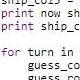• 0

# write stdout using write_csv() ...## Questiontarifa

dear Fellow Coder on Neowin

im using bash to pipe the gathered data through an Rscript like so:

`cat random.csv | Rscript test.R arg >| delete.csv`

My aim is to use the R package readr to both read stdin and write stdout. what is aimed:  I found the answer to stdin here.

```test.R

#!/usr/bin/Rscript

args  <- commandArgs(trailingOnly = TRUE)

write_csv(df.in, path = stdout())```

My investigations gave back the foolowing:

There is a format_csv function for that in readr. Use this instead of write_csv:

`cat(format_csv(df.in))`

we also can use write.table:

```write.table(x, file = "foo.csv", sep = ",", col.names = NA,

qmethod = "double")```

i found the following interesting help page: https://stat.ethz.ch/R-manual/R-devel/library/utils/html/write.table.html

```import requests
from bs4 import BeautifulSoup
import pandas as pd

def Main(urls):
with requests.Session() as req:
allin = []
for url in urls:
r = req.get(url)
soup = BeautifulSoup(r.content, 'html.parser')
target = soup.find(
"dl", class_="c-description-list c-description-list--striped")
names = [item.text for item in target.findAll("dt")]
names.append("url")
data = [item.get_text(strip=True) for item in target.findAll("dd")]
data.append(url)
allin.append(data)
df = pd.DataFrame(allin, columns=names)
df.to_csv("data.csv", index=False, encoding="utf-8")

Main(urls)```
` `

the question is. i want to write the results of the parser script to stdout . - i do not want to write it into the data-file.

## Recommended Posts

• 0+virtorio

According to this:

"to_csv()" returns a string if no file path/object is specified, so you should be able to print the result to stdout.